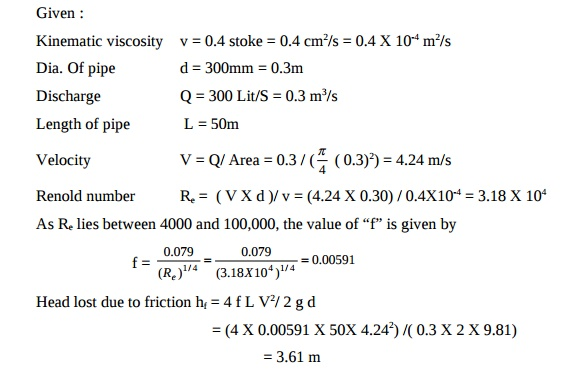Home | | Mechanics of Fluids | Mechanics Of Fluids- Boundary Layer: Introduction

# Mechanics Of Fluids- Boundary Layer: Introduction

Hydraulic gradient line: It is defined as the line which gives the sum of pressure head ( P/?g) and datum head (z) of a flowing fluid in a pipe with respect to some reference line or is the line which is obtained by joining the top of all vertical ordinates, showing the pressure head ( P/?g) of a pipe from the center of the pipe.

Boundary Layer

Introduction

It is defined as the line which gives the sum of pressure head ( P/?g) and datum head (z) of a flowing fluid in a pipe with respect to some reference line or is the line which is obtained by joining the top of all vertical ordinates, showing the pressure head ( P/?g) of a pipe from the center of the pipe. It is briefly written as H.G.L

Major energy loss and minor energy loss in pipe

The loss of head or energy due to friction in pipe is known as major loss while the loss of energy due to change of velocity of the flowing fluid in magnitude or direction is called minor loss of energy.

Total Energy line

It is defined as the line, which gives sum of pressure head, datum head and kinetic head of a flowing fluid in a pipe with respect to some reference line.

Equivalent pipeline

An Equivalent pipe is defined as the pipe of uniform diameter having loss of head and discharge of a compound pipe consisting of several pipes of different lengths and diameters.

Water Hammer in pipes.

In a long pipe, when the flowing water is suddenly brought to rest by closing the valve or by any similar cause, there will be a sudden rise in pressure due to the momentum of water being destroyed. A pressure wave is transmitted along the pipe. A sudden rise in pressure has the effect of hammering action on the walls of the pipe. This phenomenon of rise in pressure is known as water hammer or hammer blow.

The boundary layer is called laminar, if the Renolds number of the flow is defined as Re = U x X / v is less than 3X105

If the Renolds number is more than 5X105, the boundary layer is called turbulent boundary.

Where, U = Free stream velocity of flow X = Distance from leading edge v = Kinematic viscosity of fluid

Chezy's formula.

Chezy's formula is generally used for the flow through open channel.

V =C.Rt(mi)

Where , C = chezy's constant, m = hydraulic mean depth and i = hf/ L.

Problem 1

A crude of oil of kinematic viscosity of 0.4 stoke is flowing through a pipe of diameter 300mm at the rate of 300 litres/sec. find the head lost due to friction for a length of 50m of the pipe.Problem 2

Find the type of flow of an oil of relative density 0.9 and dynamic viscosity 20 poise, flowing through a pipe of diameter 20 cm and giving a discharge of 10 lps. Solution :

s = relative density = Specific gravity = 0.9 m = Dynamic viscosity = 20 poise = 2 Ns/m2.

Dia of pipe D = 0.2 m; Discharge Q = 10 lps = (10 / 1000) m3/s; Q = AV. So V = Q / A = [10 / (1000 X ( p4 ( 0.2)2) )] = 0.3183 m/s.

Kinematic viscosity = v = m / ? = [ 2 / (0.9X1000)] = 2.222X10-3 m2 / s. Reynolds number Re = VD / v

Re = [0.3183 X 0.2 / 2.222X10-3] = 28.647; Since Re ( 28.647) < 2000,

It is Laminar flow.

Formula for finding the loss of head due to entrance of pipe hi

hi  = 0.5 ( V2 / 2g)

Formula to find the Efficiency of power transmission through pipes

n = ( H - hf) / H

where, H = total head at inlet of pipe. hf = head lost due to friction

Problem 3

Hydro dynamically smooth pipe carries water at the rate of 300 lit/s at 20oC (r = 1000 kg/m3, n = 10-6 m2/s) with a head loss of 3m in 100m length of pipe.

Determine the pipe diameter. Use f = 0.0032 + (0.221)/ (Re)0.237 equation for f where hf = ( fXLXV2)/ 2gd and Re = (rVD/m)Study Material, Lecturing Notes, Assignment, Reference, Wiki description explanation, brief detail
Civil : Mechanics Of Fluids : Boundary Layer : Mechanics Of Fluids- Boundary Layer: Introduction |